Search by Topic

Resources tagged with Addition & subtraction similar to Round and Round and Round:

Filter by: Content type:
Age range:
Challenge level:

There are 60 results

Broad Topics > Calculations and Numerical Methods > Addition & subtraction3388

Age 11 to 14 Challenge Level:

Using some or all of the operations of addition, subtraction, multiplication and division and using the digits 3, 3, 8 and 8 each once and only once make an expression equal to 24.As Easy as 1,2,3

Age 11 to 14 Challenge Level:

When I type a sequence of letters my calculator gives the product of all the numbers in the corresponding memories. What numbers should I store so that when I type 'ONE' it returns 1, and when I type. . . .Like Powers

Age 11 to 14 Challenge Level:

Investigate $1^n + 19^n + 20^n + 51^n + 57^n + 80^n + 82^n$ and $2^n + 12^n + 31^n + 40^n + 69^n + 71^n + 85^n$ for different values of n.Subtraction Surprise

Age 7 to 14 Challenge Level:

Try out some calculations. Are you surprised by the results?The Patent Solution

Age 11 to 14 Challenge Level:

A combination mechanism for a safe comprises thirty-two tumblers numbered from one to thirty-two in such a way that the numbers in each wheel total 132... Could you open the safe?Multiply the Addition Square

Age 11 to 14 Challenge Level:

If you take a three by three square on a 1-10 addition square and multiply the diagonally opposite numbers together, what is the difference between these products. Why?Arrange the Digits

Age 11 to 14 Challenge Level:

Can you arrange the digits 1,2,3,4,5,6,7,8,9 into three 3-digit numbers such that their total is close to 1500?Alphabet Soup

Age 11 to 14 Challenge Level:

This challenge is to make up YOUR OWN alphanumeric. Each letter represents a digit and where the same letter appears more than once it must represent the same digit each time.Chameleons

Age 11 to 14 Challenge Level:

Whenever two chameleons of different colours meet they change colour to the third colour. Describe the shortest sequence of meetings in which all the chameleons change to green if you start with 12. . . .Largest Number

Age 11 to 14 Challenge Level:

What is the largest number you can make using the three digits 2, 3 and 4 in any way you like, using any operations you like? You can only use each digit once.Digit Sum

Age 11 to 14 Challenge Level:

What is the sum of all the digits in all the integers from one to one million?Adding and Subtracting Positive and Negative Numbers

Age 11 to 14

How can we help students make sense of addition and subtraction of negative numbers?Have You Got It?

Age 11 to 14 Challenge Level:

Can you explain the strategy for winning this game with any target?Kids

Age 11 to 14 Challenge Level:

Find the numbers in this sumJugs of Wine

Age 11 to 14 Challenge Level:

You have four jugs of 9, 7, 4 and 2 litres capacity. The 9 litre jug is full of wine, the others are empty. Can you divide the wine into three equal quantities?Pairs

Age 11 to 14 Challenge Level:

Ann thought of 5 numbers and told Bob all the sums that could be made by adding the numbers in pairs. The list of sums is 6, 7, 8, 8, 9, 9, 10,10, 11, 12. Help Bob to find out which numbers Ann was. . . .2010: A Year of Investigations

Age 5 to 14

This article for teachers suggests ideas for activities built around 10 and 2010.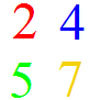And So on and So On

Age 11 to 14 Challenge Level:

If you wrote all the possible four digit numbers made by using each of the digits 2, 4, 5, 7 once, what would they add up to?Countdown

Age 7 to 14 Challenge Level:

Here is a chance to play a version of the classic Countdown Game.Score

Age 11 to 14 Challenge Level:

There are exactly 3 ways to add 4 odd numbers to get 10. Find all the ways of adding 8 odd numbers to get 20. To be sure of getting all the solutions you will need to be systematic. What about. . . .Making Sense of Positives and Negatives

Age 11 to 14

This article suggests some ways of making sense of calculations involving positive and negative numbers.Got it for Two

Age 7 to 14 Challenge Level:

Got It game for an adult and child. How can you play so that you know you will always win?Got it Article

Age 7 to 14

This article gives you a few ideas for understanding the Got It! game and how you might find a winning strategy.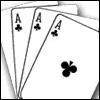Amazing Card Trick

Age 11 to 14 Challenge Level:

How is it possible to predict the card?Pyramids

Age 11 to 14 Challenge Level:

What are the missing numbers in the pyramids?Weights

Age 11 to 14 Challenge Level:

Different combinations of the weights available allow you to make different totals. Which totals can you make?Football Sum

Age 11 to 14 Challenge Level:

Find the values of the nine letters in the sum: FOOT + BALL = GAMEMagic Squares for Special Occasions

Age 11 to 16

This article explains how to make your own magic square to mark a special occasion with the special date of your choice on the top line.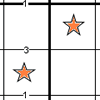Constellation Sudoku

Age 14 to 18 Challenge Level:

Special clue numbers related to the difference between numbers in two adjacent cells and values of the stars in the "constellation" make this a doubly interesting problem.Eleven

Age 11 to 14 Challenge Level:

Replace each letter with a digit to make this addition correct.Aba

Age 11 to 14 Challenge Level:

In the following sum the letters A, B, C, D, E and F stand for six distinct digits. Find all the ways of replacing the letters with digits so that the arithmetic is correct.Tis Unique

Age 11 to 14 Challenge Level:

This addition sum uses all ten digits 0, 1, 2...9 exactly once. Find the sum and show that the one you give is the only possibility.Crossed Ends

Age 11 to 14 Challenge Level:

Crosses can be drawn on number grids of various sizes. What do you notice when you add opposite ends?Number Daisy

Age 11 to 14 Challenge Level:

Can you find six numbers to go in the Daisy from which you can make all the numbers from 1 to a number bigger than 25?Two and Two

Age 11 to 14 Challenge Level:

How many solutions can you find to this sum? Each of the different letters stands for a different number.Cayley

Age 11 to 14 Challenge Level:

The letters in the following addition sum represent the digits 1 ... 9. If A=3 and D=2, what number is represented by "CAYLEY"?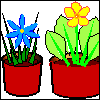More Children and Plants

Age 7 to 14 Challenge Level:

This challenge extends the Plants investigation so now four or more children are involved.Pair Sums

Age 11 to 14 Challenge Level:

Five numbers added together in pairs produce: 0, 2, 4, 4, 6, 8, 9, 11, 13, 15 What are the five numbers?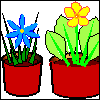More Plant Spaces

Age 7 to 14 Challenge Level:

This challenging activity involves finding different ways to distribute fifteen items among four sets, when the sets must include three, four, five and six items.Always the Same

Age 11 to 14 Challenge Level:

Arrange the numbers 1 to 16 into a 4 by 4 array. Choose a number. Cross out the numbers on the same row and column. Repeat this process. Add up you four numbers. Why do they always add up to 34?Making Maths: Double-sided Magic Square

Age 7 to 14 Challenge Level:

Make your own double-sided magic square. But can you complete both sides once you've made the pieces?Top-heavy Pyramids

Age 11 to 14 Challenge Level:

Use the numbers in the box below to make the base of a top-heavy pyramid whose top number is 200.Nice or Nasty

Age 7 to 14 Challenge Level:

There are nasty versions of this dice game but we'll start with the nice ones...Twenty20

Age 7 to 16 Challenge Level:

Fancy a game of cricket? Here is a mathematical version you can play indoors without breaking any windows.Countdown Fractions

Age 11 to 16 Challenge Level:

Here is a chance to play a fractions version of the classic Countdown Game.Nice or Nasty for Two

Age 7 to 14 Challenge Level:

Some Games That May Be Nice or Nasty for an adult and child. Use your knowledge of place value to beat your opponent.Postage

Age 14 to 16 Challenge Level:

The country Sixtania prints postage stamps with only three values 6 lucres, 10 lucres and 15 lucres (where the currency is in lucres).Which values cannot be made up with combinations of these postage. . . .Pole Star Sudoku 2

Age 11 to 16 Challenge Level:

This Sudoku, based on differences. Using the one clue number can you find the solution?Number Pyramids

Age 11 to 14 Challenge Level:

Try entering different sets of numbers in the number pyramids. How does the total at the top change?Magic Squares

Age 14 to 18

An account of some magic squares and their properties and and how to construct them for yourself.